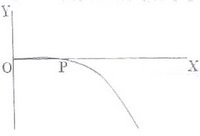## Monday, October 23, 2006

### Magnetic Force on Moving Charges

"You may never know what results come of your actions, but if you do nothing, there will be no results."
– Mahatma Gandhi

Most of you might be remembering the expression for the magnetic force ‘F’ on a charge ‘q’ moving with velocity ‘v’ in a magnetic field of flux density ‘B’:
F = qvB sinθ where θ is the angle between v and B. Retain the order qvB. It helps you to remember this expression in its vector form:
F = qv×B
The vector form gives you the magnitude (qvB sinθ) of the force and its direction which is along the direction of the cross product vector v×B. You should remember that the direction of v is the direction of motion of a positive charge. If you have a negatively charged particle such as an electron, you should reverse the direction of v to get the direction of the force.
If there is an electric field (E) also in the region, the net force on the charge is given by
F = q(v×B + E).
This is the Lorentz force equation.
Let us consider the following MCQ which appeared in the IIT-JEE Screening 2003 question paper:
For a positively charged particle moving in XY plane initially along the X-axis, there is a sudden change in its path due to the presence of electric and/or magnetic fields beyond P. The curved path is shown in the XY plane and is foundto be non-circular.Which one of the following
combinations is possible? (i,j,k are unit
vectors along X,Y,Z directions)
(a) E = 0; B =bi + c
(b) E = ai; B =ck + ai
(c) E = 0; B =cj + bk(d) E = ai; B =ck + bj
As the curved path is confined to the XY plane,
the component of magnetic field effective in
deflecting the particle is the Z-component only.
The other component should be the X-component since the X-component cannot deflect the charged particle proceeding along the X-direction. There has to be an electric field since the path of the particle is non-circular. All these conditions are satisfied by option (b).
Now consider the following multiple choice questions:
(1)An electron is projected horizontally from south to north in a uniform horizontal magnetic field acting from west to east. The direction along which it will be deflected by this magnetic field is
(a) vertically upwards

(b) vertically downwards
(c) northwards
(d) southwards
(e) eastwards
Since the electron is negatively charged, the direction of its velocity is to be reversed for finding the direction of the cross product vector v
×B. The correct option therefore is (a). You can use Fleming’s left hand rule (motor rule) also for finding the direction of the magnetic force. But, when you apply the rule, remember that the direction of the conventional current is that of positive charge.
(2) An electron enters a uniform magnetic field of flux density B=2i+6j-√8 k with a velocity V=i+3j-√2 k where i,j and k are unit vectors along the X, Y and Z directions respectively. Then
(a) both speed and path will change

(b) speed alone will change
(c) the path will become circular
(d) the path will become helical (e) neither speed nor path will change
The vectors B and V are parallel as the components of B are twice the components of V. So the magnetic force on the electron is zero and the correct option is (e).
Generally, in problems of the above type, you will have to find the angle between the vectors V and B using cosθ = V.B/ VB. In the present case we have, cosθ = (2+18+4)/ √(12×48) = 1 so that θ= zero. If in a similar problem, the value of θ works out to be 90˚(if cos
θ works out to be zero), the correct option would be (c). If the value of θ were neither zero nor 90˚, the path would be helical and the correct option would be (d).
(3) Doubly ionized atoms X and Y of two different elements are accelerated through the same potential difference. On entering a uniform magnetic field, they describe circular paths of radii R1 and R2. The masses of X and Y are in the ratio
(a) R1/R2 (b) √(R1/R2) (c) √(R2/R1) (d) (R2/R1)2 (e) (R1/R2)2

Since both atoms are doubly ionized, they have the same charge ‘q’ and since they are accelerated by the same potential difference ‘V’, they have the same kinetic energy, qV. If m1 and m2 are the masses and v1 and v2 are the velocities of X and Y respectively, we have, ½ m1v12 = ½ m2v22 from which m1/m2 = v22/v12 = [qBR2/m2]2/ [qBR1/m1]2, on substituting for the velocities from the centripetal force equation, mv2/R = qvB.
Therefore, m1/m2 = (R1/R2)2, given by option (e).